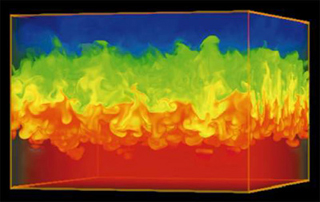MIT# [100% Off] NUMERICAL MARINE HYDRODYNAMICS (13.024) Free Course Coupon

Updated: by ghatfanmerie

Click to get the course

8.0 hr
English
Free
Get the coupon in the end of description.

### Description

This course is an introduction to numerical methods: interpolation, differentiation, integration, and systems of linear equations. It covers the solution of differential equations by numerical integration, as well as partial differential equations of inviscid hydrodynamics: finite difference methods, boundary integral equation panel methods. Also addressed are introductory numerical lifting surface computations, fast Fourier transforms, the numerical representation of deterministic and random sea waves, as well as integral boundary layer equations and numerical solutions.
If the coupon is not opening, disable Adblock, or try another browser.